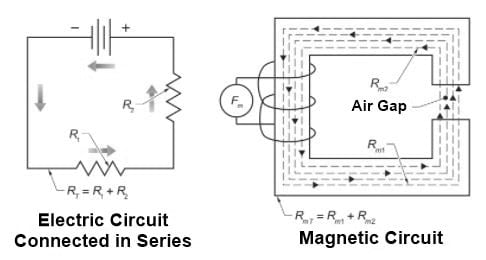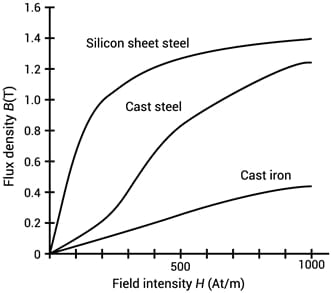Technical Article

# Magnetic Circuit Properties: Understanding Reluctance

September 15, 2021 by Alex Roderick

## The property of a magnetic circuit that opposes magnetic lines of force is known as reluctance.

The magnetic material's reluctance varies with flux density.  The unit for magnetic reluctance is ampere-turns / Weber (AT / Wb) or inverse Henry (H-1). Reluctance in magnetic circuits is similar to resistance in electrical circuits. Reluctance is calculated by applying the following formula:

${{R}_{m}}=\frac{{{F}_{m}}}{\phi }$

where

$$R_{m}$$ = reluctance in ampere-turns per weber

$$F_{m}$$ = magnetomotive force (MMF) in ampere-turns

$$\phi$$ = the magnetic flux in webers

Example: What is the reluctance in a circuit with a magnetic flux of 1 mWb and a total circuit mmf of 1750 ampere-turns?

${{R}_{m}}=\frac{{{F}_{m}}}{\phi }=\frac{1750\text{ }Ampere-turns}{1\text{ }mWb}=1.75 \times 10^{6} \frac{AT}{Wb}$

Like resistance, reluctance changes in direct proportion to the core material's length and in inverse proportion to its area and permeability. Permeability (μ) is a material's ability to carry magnetic lines of force.

Although the reluctance measured of a coil with an air gap is linear, reluctance measured using a magnetic substance is nonlinear. A permeability chart is used to obtain reluctance values.  Air exhibits reluctance 50 times to 5000 times greater than that of magnetic materials. Nonmagnetic materials, such as paper and wood, have about the same value of reluctance (within ±1%) as air. Magnetic circuits that combine a magnetic material with an air gap are common. In a magnetic circuit, reluctances add together in the same way as resistance in an electric circuit connected in series does (see Figure 1).##### Figure 1. In a magnetic circuit, reluctances add together in the same way as resistance in an electric circuit connected in series does.

Reluctance in a magnetic circuit with several paths in series can be calculated by applying the following formula:

${{R}_{mT}}={{R}_{m1}}+{{R}_{m2}}+\cdots +{{R}_{mN}}$

where

$$R_{mT}$$ = total circuit reluctance (in ampere-turns per weber)

$$R_{m1}$$ = path 1 reluctance (in ampere-turns per weber)

$$R_{m2}$$ = path 2 reluctance (in ampere-turns per weber)

$$R_{mN}$$ = path N reluctance (in ampere-turns per weber)

Example: What is the total circuit reluctance in a magnetic circuit that has 1.5×106 AT / Wb, 2×106 AT / Wb, and 3×106 AT / Wb rels in series?

\begin{align}& {{R}_{mT}}={{R}_{m1}}+{{R}_{m2}}+{{R}_{m3}} \\ & {{R}_{mT}}=50\times {{10}^{-6}}+30\times {{10}^{-6}}+20\times {{10}^{-6}} \\ & {{R}_{mT}}=0.0001\text{ }rels \\ \end{align}

\begin{align}& {{R}_{mT}}={{R}_{m1}}+{{R}_{m2}}+{{R}_{m3}} \\ & {{R}_{mT}}=1.5\times {{10}^{6}}+2\times {{10}^{6}}+3\times {{10}^{6}} \\ & {{R}_{mT}}=6.5\times {{10}^{6}}\text{ }\frac{AT}{Wb} \\ \end{align}

## Magnetizing Force Intensity

The magnetizing force intensity measures the magnetic strength per unit length. The higher the current, the stronger the produced magnetic fields. The intensity of magnetizing force is calculated by applying the following procedure:

1.       Calculate magnetomotive force (mmf). The mmf is calculated by applying the following formula:

${{F}_{m}}= I\times N$

2.       Calculate the intensity of magnetizing force.

$H=\frac{{{F}_{m}}}{l}$

Where

$$F_{m}$$ = total mmf in ampere-turns

$$I$$ = current (in A)

$$N$$ = number of turns

$$H$$ = intensity of magnetizing force in ampere-turns per meter

$$l$$ = length in meters

Example: What is the intensity of magnetizing force for a conductor carrying 100 mA with 1000 turns over a 10 cm length?

Calculate the mmf.

${{F}_{m}}=I\times N=0.100\text{ }A\text{ }\times \text{ }1000\text{ }turns=100\text{ } \text{ }ampere-turns$

Calculate the intensity of the magnetizing force.

$H=\frac{{{F}_{m}}}{l}=\frac{100}{0.1}=1000\text{ }\frac{ampere-turns}{meter}$

The magnetic intensity from a conductor can also be measured in Oersted. The magnetic intensity at 1 cm from the center of a conductor carrying 5 A is 1 Oe. The magnetic field intensity of a conductor is calculated by applying the following formula:

$H=\frac{I}{5\times r}$

where

$$H$$ = magnetic field intensity (in Oe)

$$I$$ = current (in A)

$$5$$ = constant

$$r$$ = distance from conductor center (in cm)

Example: What is the magnetic field intensity (in Oersted) at a distance of 15 cm from the center of a straight conductor carrying 2.25 A?

$H=\frac{I}{5\times r}=\frac{2.25}{5\times 15}=0.03\text{ Oe}$

Magnetic field intensity at the center of a flat circular coil with current flowing through it is calculated by applying the following formula:

$H=\frac{\pi \times N\times I}{5\times r}$

where

$$H$$ = magnetic field intensity (in Oe)

$$\pi$$ = ratio of coil circumference to diameter (3.14)

$$N$$ = number of turns

$$I$$ = current (in A)

$$r$$ = radius of turns (in cm)

Example: What is the magnetic field intensity at the center of a flat circular coil of 500 turns that is 20 cm in diameter and carries 3 A?

$H=\frac{\pi \times N\times I}{5\times r}=\frac{3.14\times 500\times 3}{5\times 20}=94.2\text{ Oe}$

## What is Permeability?

Permeability (μ) is a material's ability to carry magnetic lines of force. Permeability (μ) is defined as the ratio of flux density (B) in Tesla (T) to the field intensity (H) in ampere-turns per meter. A vacuum has a permeability of 1. The permeability of air is also designated as 1 but is actually slightly higher at 1.0000004. The unit of magnetic permeability is henries per meter (H/m).

A gauss is equal to one line of force per square centimeter. Gauss (G) measures the amount of density for magnetic lines of force (flux density). The Earth's flux density is about 1 G at the surface. Flux density is calculated by applying the following formula:

$B=\frac{\phi }{A}$

where

$$B$$ = flux density in Tesla (T)

$$\phi$$ = total magnetic lines of force (total magnetic flux) in weber (Wb)

$$A$$ = total area of magnetic lines of force in m

The above equation assumes that the surface with area A is perpendicular to the field lines and the field is uniform over this area.

Example: What is the flux density of a cube that has lengths of 10 cm and magnetic lines of force of 1×10-8 Wb?

$B=\frac{\phi }{A}=\frac{10^{-8}}{0.1\times 0.1}=10^{-6}\text{ }T$

Variation in the intensity of the magnetizing force (H) applied to nonmagnetic substances results in a proportional increase or decrease in the flux density (B) and shows a linear relationship between B and H.

This relationship is not true for magnetic substances. The density of the magnetic lines of force of a magnetic substance depends on the permeability of the substance and the amount of ampere-turns per unit length. The permeability curve of a ferromagnetic material is nonlinear. Figure 2 shows the magnetization curves for three different types of material. The flux density rises quickly at the beginning of the magnetizing process and at a greatly reduced rate afterward. At some point, a magnetic saturation occurs. Magnetic saturation is the point at which a further increase in current does not cause a further increase in the number of magnetic lines of force.##### Figure 2. The magnetization curves for soft iron, soft cast steel, and cast iron show a greater flux density for soft iron than for soft cast steel or cast iron.

A paramagnetic substance is one that has a constant relative permeability of slightly more than 1. A paramagnetic substance has a greater ability to be magnetized than air but less ability than a ferromagnetic material. A diamagnetic substance is one that is repelled by either pole of a magnet and has a constant relative permeability of slightly less than 1. A ferromagnetic substance is one with a permeability much much greater than one. A ferromagnetic material does not have a constant relative permeability.

A simple process determines whether a substance is paramagnetic, diamagnetic, or ferromagnetic. If the substance is suspended between the poles of a strong magnet and it aligns itself so that its longer dimension is in line with the field, then it is either ferromagnetic or paramagnetic. If the substance aligns itself so that it assumes a position perpendicular to the magnetic field, then the substance is diamagnetic. Permeability is calculated by applying the following formula:

$\mu =\frac{B}{H}$

where

$$μ$$ = permeability in henries per meter (H/m)

$$B$$ = flux density in Tesla (T)

$$H$$ = magnetizing force in ampere-turns per meter

Example: What is the permeability of a substance that has a field intensity of 2000 ampere-turns per meter and a flux density of 12.5 T?

$\mu =\frac{B}{H}=\frac{12.5}{2000}=0.00625\text{ }{H}/{m}$

Featured Image used courtesy of Britannica